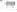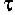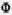### FREE CONVECTION 2

#### OPTIMIZATION OF PULSATING HEATING IN NATURAL AND FORCED CONVECTION

J. A. Jones Professor of Mechanical Engineering
Duke University
Durham, NC 27708-0300, USA

This is a review of recent theoretical, numerical and experimental studies of natural and forced convection heat transfer from surfaces with intermittent (on & off) heat flux. Scale analyses predict that in each configuration there is an optimal heat pulse interval (or frequency) that optimizes the overall thermal conductance between the heater and the external fluid. This feature was verified and determined more accurately by complete numerical simulations and experiments with water in natural convection, and air in forced convection. In natural convection, the heater configurations optimized experimentally were the vertical cylinder, which approximates the vertical plane wall, and the horizontal cylinder. In forced convection, the tested configuration was the single cylinder in crossflow.

#### QUASI STEADY-STATE NATURAL CONVECTIVE HEAT TRANSFER FROM ISOTHERMAL CIRCULAR FLAT SURFACES

W. M. Lewandowski, P. Kubski, S. Szymanski, H. Bieszk, T. Wilczewski and T. Seramak
Technical University of Gdansk
Department of Apparatus and Chemical Machinery
ul.G.Narutowicza 11/12, 80-952 Gdansk, Poland

Theoretical considerations on quasi steady-state convective heat transfer from isothermal circular flat surface have been presented. The physical model of this phenomenon consists of an isothermal cone of inclination angle (= 0 - circular horizontal plate) to (=/2 - vertical cylinder). The temperature of the surface is constant (Tw = const) in the contrary the temperature of ambient fluid which is changing according to relation (T* = T+a.sin). On the base of Navier-Stokes equations, assuming the parabolic temperature profile in the boundary layer, the velocity profile tangent to the surface has been calculated. Introduction of the mean velocity value in the boundary layer into the balance of energy and mass equations and comparison with the Newton equation leads to the dependence describing the boundary layer thickness. Next the relation of Nusselt and Rayleigh numbers, including a function expressing the influence of the inclination angle () on the heat transfer process has been derived. Obtained solution describes the natural convective heat transfer process for three characteristic cases of the circular flat surface:

For the boundary cases=/2 (vertical cylinder) and=0 (circular horizontal plate) the solution describing convective heat transfer intensity is:

NuR=0.669.RaR1/4.1/4 for=/2 and NuR=0.932.RaR1/5.1/5 for=0,
For the case (0 <</2) (cones) the solution has the form:

NuR=1.680.1/4.RaR1/4.1/4
where () is a function of the inclination angle () of the generating line of the rotational surface to the base of radius (R) and () is a coefficient of the temperature fluctuation.

#### FINITE ELEMENT MODELLING OF INCOMPRESSIBLE THERMO-CONVECTIVE FLOWS

P. Arlabosse, M. Medale, M. Jaeger and R. Martin
IUSTI, University of Provence, 13397 Marseille Cedex 20, France

Thermocapillary flow induced by a gas bubble in a silicone oil layer submitted to a vertical temperature gradient is investigated numerically. A bidimensional model based on finite element method has been developed. It enables simulations of laminar flows induced by a surface tension gradient. Different configurations are selected: Marangoni convection acting alone, natural convection type Rayleigh Benard, Marangoni convection acting against gravity... The velocity and temperature fields are computed in a vertical cross section. The combined effects of gravity and thermocapillarity are studied. Numerical results are compared with experimental measurements obtained in a previous experiment.

#### CONVECTION RESONANCE AND HEAT TRANSFER ENHANCEMENT OF PERIODICALLY HEATED FLUID ENCLOSURES

José L. Lage and Bogdan V. Antohe
Mechanical Engineering Department
Southern Methodist University
Dallas, TX 75275-0337
USA

We report on the theoretical and numerical analysis of the heat and momentum transport inside a rectangular enclosure filled with fluid, or with a fully saturated porous medium, under time-periodic horizontal heating. Numerical simulations indicate that the natural convection activity within the enclosure peaks at several discrete frequencies, with the climax attained at a heating frequency referred to as resonance frequency. We tested a general theory for predicting this resonance frequency against numerical results considering the Prandtl number effect. Results of experiments considering a fluid system under time periodic horizontal heating are also reported. Heating period from 32 to 1600 seconds, and time averaged heat-flux from 355 to 1420 W/m2, were considered. For high heat fluxes, the oscillatory heating yielded a time averaged heat transfer coefficient 20 percent higher than obtained with steady heating at a critical pulsating frequency, in good agreement with the numerical and theoretical predictions.